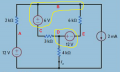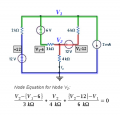# How do i use supernode in this circuit?

#### vlagvlag

Joined Nov 5, 2016
9
https://s11.postimg.org/tctbopgvn/Capture.jpg

So basically im supposed to use source transformation to solve this problem , so i managed to transform all voltage sources into current sources with resistors in parallel and ended up with 2,6 k resisors in parralel with a 4 mA source so Io= 1mA , but then i tried to solve this problem using nodal analysis and i cant seem to know what to do if i pick ground node to be the bottom node , then i'd have two nodes in this circuit, right?, i cant seem to figure out how to deal with the 2 adjacent voltage sources, i know supernodes must be involved but i cant seem to be able to right their equation , any help is appreciated and thanks in advance.

#### MrAl

Joined Jun 17, 2014
8,543
Hi,

One way to look at it is that you both short the source out and open circuit the source.

You short the source from v1 to v2 out in order to see that a node v1 is the same node as v2, but you open circuit the source to see that they are both still different variables. So short it out, then write the equations, but keep v1 and v2 variables separate.

We can do a simple example if you like.

#### WBahn

Joined Mar 31, 2012
26,398
https://s11.postimg.org/tctbopgvn/Capture.jpg

So basically im supposed to use source transformation to solve this problem , so i managed to transform all voltage sources into current sources with resistors in parallel and ended up with 2,6 k resisors in parralel with a 4 mA source so Io= 1mA , but then i tried to solve this problem using nodal analysis and i cant seem to know what to do if i pick ground node to be the bottom node , then i'd have two nodes in this circuit, right?, i cant seem to figure out how to deal with the 2 adjacent voltage sources, i know supernodes must be involved but i cant seem to be able to right their equation , any help is appreciated and thanks in advance.
A picture would be worth a thousand words here.

Are you trying to do nodal analysis on the original problem, or on the circuit after you transformed the voltage sources into current sources. I have to assume the former since you mention having problems with adjacent voltage sources. But then you say you'd have two nodes -- what are the two nodes that you are referring to? Which of the three voltage sources are you considering to be "adjacent"? The two 12 V sources? If so, why isn't the 6 V source causing you grief?

#### vlagvlag

Joined Nov 5, 2016
9
im trying to do nodal analysis on the original problem, i consider the 12v and the 6v sources in the middle to be adjacent , and i take the two nodes , the first is between the 3k resisor and the 12v source, the other is between the 6v source and the 6k resistor , i cant seem to be able to write supernode equation when there is a resistor connected to the source in a supernode

#### WBahn

Joined Mar 31, 2012
26,398
Again, a picture is worth a thousand words. Please provide one!

What are you defining as your supernode(s)?

Use Paint (or any other free, simple-to-use image editor) and provide node labels and circle your supernodes.

The provide your best attempt at writing your node equations -- they don't have to be correct, but it is very hard to see where you are going wrong when you won't show where you went in the first place.

#### WBahn

Joined Mar 31, 2012
26,398
ok so i circled what i think is the supernodes, and labeled the two nodes https://s16.postimg.org/xpvtfirw5/Captur1e.jpg
Your problem is becoming a lot clearer. Both of your supernodes include the top node. They also both include a component other than a voltage source. That makes things a lot harder (as you have been finding out).

Your supernodes should be constructed by picking a node and then encompassing any nodes connected to it by an ideal voltage source. For instance:You now apply nodal analysis to each node and/or supernode just as you normally would, namely by summing up all the currents leaving it in terms of the voltages at the node terminals and the voltages on the other side of the adjacent components. You also need to include supplementary equations to relate the terminal node voltages of the supernodes.

#### vlagvlag

Joined Nov 5, 2016
9
so that means i have to solve for four unknowns Vb,Vc,Vd,Ve ?

#### WBahn

Joined Mar 31, 2012
26,398
Yes, but two of those are trivial:

•vlagvlag

#### RBR1317

Joined Nov 13, 2010
674
My first rule for supernode analysis is to color code the supernodes. Second rule is to annotate the diagram with the supernode offset voltages (as shown in grey on the diagram). This makes it easy to write the node equations directly from the diagram. For example, the node equation for V2 is shown here. How can you tell it is for node V2? The first clue is that every term in the summation begins with "V2".#### MrAl

Joined Jun 17, 2014
8,543
Hi,

Here are two examples. Most notable is that all the nodes connected to source E1 are included in the first equation.

Code:
[FONT=Courier New]EXAMPLE 1[/FONT]

[FONT=Courier New]EXAMPLE 1

[v2]     [v1]
+---E1---+
|        |
R1       R2
|        |
GND      GND

v1/R2+v2/R1=0
v1-v2=E1

EXAMPLE 2

[v3]     [v1]     [v2]
+---E1---+---R3---+
|        |        |
R1       R2       R4
|        |        |
GND      GND      GND

v1/R2+v1/R3-v2/R3+v3/R1=0
v1/R3+v2/R3+v2/R4+0=0
v1-v3=E1

[/FONT]

#### vlagvlag

Joined Nov 5, 2016
9
okey so i solved the 4 equations to get vd= 4v , so that means that Io = 1 mA , thank you all for the help

#### MrAl

Joined Jun 17, 2014
8,543
okey so i solved the 4 equations to get vd= 4v , so that means that Io = 1 mA , thank you all for the help

Hi,

I verified that the voltage is 4 volts also so you got it now#### MrAl

Joined Jun 17, 2014
8,543
Hi again,

Here is another example if you really want some practice...

Code:
[FONT=Courier New]
+                 +
8---E2---7---R4---6---E4---5
|        |        |        |
R1       R3       R5       R7
|        |     +  |        |
4---R2---3---E3---2---R6---1--Vo1
+|        |        |        |
E1       R8       R9       R10
|        |        |        |
GND      GND      GND      GND

R1=1k, R2=2k, R3=3k, ..., R10=10k
E1=1v, E2=2v, E3=3v, E4=4  (positive polarity terminals marked)
Nodes numbers shown at junctions.

Solutions for each node:
v1=84330/23407,v2=84501/23407,v3=14280/23407,v4=1,v5=286323/46814,v6=99067/46814,v7=110355/46814,v8=16727/46814

float solutions for v1 through v8:
3.6027684026146,3.61007390951425,0.6100739095143,1,6.11618319306191,2.11618319306191,2.3573076430128,0.3573076430128

#### The Electrician

Joined Oct 9, 2007
2,866
Just because the symbolic solution involves many terms doesn't mean that finding it isn't trivial when using modern mathematical software, which you did.

My HP50G calculator found the numerical solution in less than 1 second. Finding the symbolic solution is not of much use other than to demonstrate the power of mathematical software. My calculator could find the symbolic solution, but it would probably take the poor little thing a half hour.

For extra credit, find the current in each of the voltage sources

•anhnha

#### MrAl

Joined Jun 17, 2014
8,543
It may be good practice for writing node equations; however, solving those equations is not a trivial pursuit - as the attached pdf document demonstrates.
View attachment 114919
Hello again,

Yes when you do it by hand as Electrician pointed out, but for this many i use software too. It also makes it easier if we have the actual values from the start, such as R1=1, R2=2, etc., as we can just use numerical values then.

What i was after was a network where there were a lot of 'super' nodes. What you did though used a different approach, which is surely good too, but does not illustrate the concept of how we usually view a super node. A super node is something we usually consider to be a short circuit while retaining the variables being used and that is what i had hoped to show, although i did not clearly show this yet unfortunately. So yes it may appear to be just an exercise in nodal analysisDont get me wrong though i dont reject your solution approach as i think it is a good one too.

I'll make my point a little more clear next, where we will start the analysis centered around nodes 3 and 2 (see my previous post).
We start by totally ignoring the source E3, and starting with node 3 we have:
Eq3: (v4-v3)/R2+(v7-v3)/R3-v3/R8=0

and again ignoring the source E3 and now writing for node 2 we have:
Eq2: (v6-v2)/R5+(v1-v2)/R6-v2/R9=0

but because E3 creates a super node, we turn those two into just one equation by adding them together (after all it's really assumed to be just one node):
Eq4=Eq3+Eq2=(v4-v3)/R2+(v7-v3)/R3-v3/R8 + (v6-v2)/R5+(v1-v2)/R6-v2/R9=0

and then of course add one more equation for the source itself:
v2-v3=E3

So all we had to do is add the two equations for the two nodes and then create that second equation for the source itself.

Hopefully i wrote this all out correctly.

I also found out you cant type a colon too close to an open parenthesis or else the editor assumes it is an emoticon as in:
Eq3: (thisthat)
Eq3thisthat)

Last edited:

#### RBR1317

Joined Nov 13, 2010
674
...but does not illustrate the concept of how we usually view a super node.
One may view a supernode as no different than a regular node and write a node equation for the supernode directly from the circuit diagram just like a regular node. My point is that getting the supernode equation can be made easier by color coding the supernode and annotating the voltage offsets directly on the diagram. When done in this manner the supernode equation will have the same basic form as a regular node equation, thereby making it easier to see when a simple mistake has been made.

But the underlying message in my first reply was: Don't expect to solve this problem by rote manipulation of algebraic formulae.

#### MrAl

Joined Jun 17, 2014
8,543
Hi,

Ok sure.

Would you care to tackle this circuit, but showing how the super node concept works (not just solving for Vo1 or the node voltages)?

Code:
[FONT=Courier New]
8---E2---7---R4---6---E6---5
|        |        |        |
R1       E3       E5       R7
|        |        |        |
4---R2---3---E4---2---R6---1--Vo1
|        |        |        |
E1       R8       R9       R10
|        |        |        |
GND      GND      GND      GND

[/FONT]

#### The Electrician

Joined Oct 9, 2007
2,866
Don't expect to solve this problem by rote manipulation of algebraic formulae.
What other way is there to solve it? What do you think Maple is doing? If ever there was an example of rote manipulation of algebraic formulae, mathematical software is it.

If what you mean is that a human being wouldn't be able to carry out that much algebra by hand without making a mistake, you should say that. That I can certainly agree with.

Is anybody going to give a try at solving for the voltage source currents?